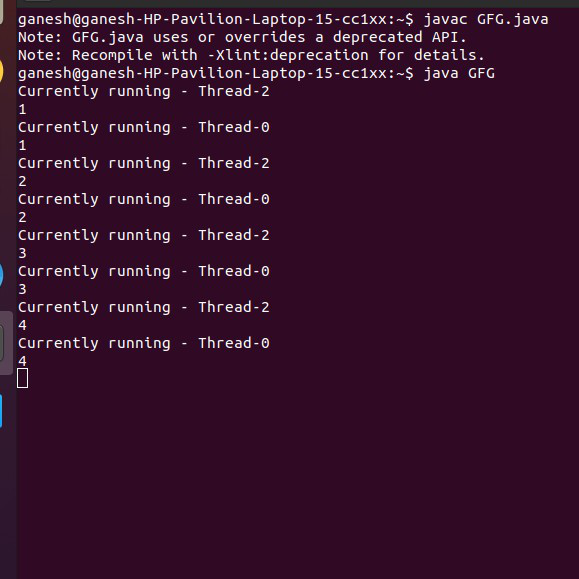# How to Temporarily Stop a Thread in Java?

• Last Updated : 24 Nov, 2020

The suspend() method of thread class puts the thread from running to waiting state. This method is employed if you would like to prevent the thread execution and begin it again when a particular event occurs. This method allows a thread to temporarily cease execution. The suspended thread is often resumed using the resume() method. If the present thread cannot modify the target thread then it’ll throw Security Exception.

Note: suspend() method is deprecated in the latest Java version.

Syntax

`public final void suspend()`

Return: Does not return any value.

Exception: Throws SecurityException If the current thread cannot modify the thread.

Example:

## Java

 `// Java program to demonstrate suspend() method``// of Thread class`` ` `import` `java.io.*;`` ` `class` `GFG ``extends` `Thread {``    ``public` `void` `run()``    ``{``        ``for` `(``int` `i = ``1``; i < ``5``; i++) {``            ``try` `{``               ` `                ``// thread to sleep for 500 milliseconds``                ``sleep(``5``);``                ``System.out.println(``                    ``"Currently running - "``                    ``+ Thread.currentThread().getName());``            ``}``            ``catch` `(InterruptedException e) {``                ``System.out.println(e);``            ``}``            ``System.out.println(i);``        ``}``    ``}``    ``public` `static` `void` `main(String args[])``    ``{``        ``// creating three threads``        ``GFG t1 = ``new` `GFG();``        ``GFG t2 = ``new` `GFG();``        ``GFG t3 = ``new` `GFG();``       ` `        ``// call run() method``        ``t1.start();``        ``t2.start();``       ` `        ``// suspend t2 thread``        ``t2.suspend();``       ` `        ``// call run() method``        ``t3.start();``    ``}``}`

OutputNote: Thread t2 can be resumed by resume() method.

`t2.resume() `

My Personal Notes arrow_drop_up